×#### Thank you for registering.

One of our academic counsellors will contact you within 1 working day.

Click to Chat

1800-1023-196

+91-120-4616500

CART 0

• 0

MY CART (5)

Use Coupon: CART20 and get 20% off on all online Study Material

ITEM
DETAILS
MRP
DISCOUNT
FINAL PRICE
Total Price: Rs.

There are no items in this cart.
Continue Shopping• Complete JEE Main/Advanced Course and Test Series
• OFFERED PRICE: Rs. 15,900
• View Details

Chapter 6: Algebraic Expressions and Identities Exercise – 6.3

Question: 1

Find products

5x2 × 4x3

Solution:

To multiply algebraic expressions, we use commutative and associative laws along with the laws of indices. However, use of these laws is subject to their applicability in the given expressions. In the present problem, to perform the multiplication, we can proceed as follows: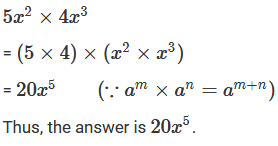Question: 2

Find products

−3a2 × 4b4

Solution:

To multiply algebraic expressions, we can use commutative and associative laws along with the law of indices, am × an = am + n, wherever applicable.

We have: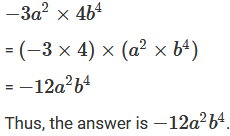Question: 3

Find products

(−5xy) × (−3x2yz)

Solution:

To multiply algebraic expressions, we can use commutative and associative laws along with the law of indices, am × an = am + n, wherever applicable.

We have: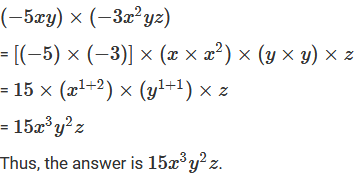Question: 4

Find products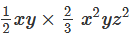Solution:

To multiply algebraic expressions, we can use commutative and associative laws along with the law of indices, am × an = am + n, wherever applicable.

We have: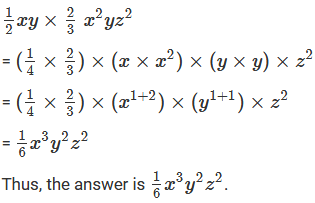Question: 5

Find productsSolution:

To multiply algebraic expressions, we can use commutative and associative laws along with the law of indices, am × an = am + n, wherever applicable.

We have: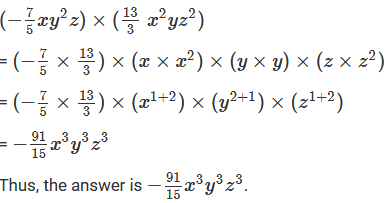Question: 6

Find productsSolution:

To multiply algebraic expressions, we can use commutative and associative laws along with the law of indices, am × an = am + n, wherever applicable.

We have: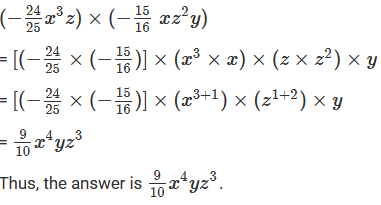Question: 7

Find products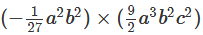Solution:

To multiply algebraic expressions, we can use commutative and associative laws along with the law of indices, am × an = am + n, wherever applicable.

We have: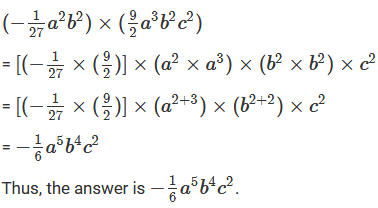Question: 8

Find products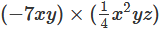Solution:

To multiply algebraic expressions, we can use commutative and associative laws along with the law of indices, am × an = am + n, wherever applicable.

We have:Question: 9

Find the products

(7ab) × (− 5ab2c) × (6abc2)

Solution:

To multiply algebraic expressions, we can use commutative and associative laws along with the law of indices, am × an = am + n, wherever applicable.

We have: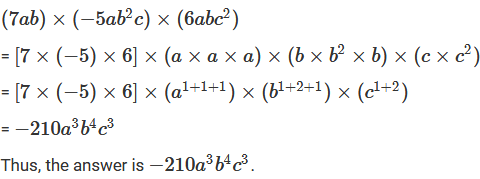Question: 10

Find the products

(−5a) × (−10a2) × (−2a3)

Solution:

To multiply algebraic expressions, we can use commutative and associative laws along with the law of indices, am × an = am + n, wherever applicable.

We have: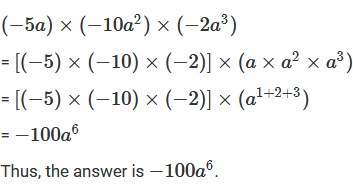Question: 11

Find the products

(−4x2) × (−6xy2) × (−3yz2)

Solution:

To multiply algebraic expressions, we can use commutative and associative laws along with the law of indices, am × an = am + n, wherever applicable.

We have: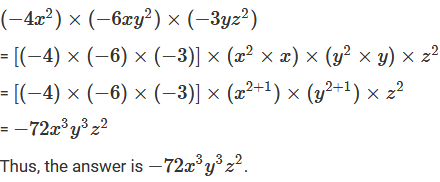Question: 12

Find the products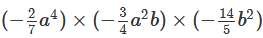Solution:

To multiply algebraic expressions, we can use commutative and associative laws along with the law of indices, am× an = am + n, wherever applicable.

We have: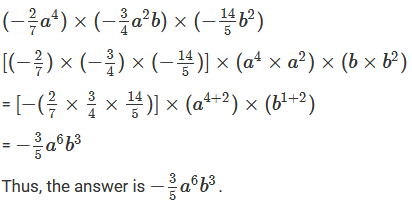Question: 13

Find the productsSolution:

To multiply algebraic expressions, we can use commutative and associative laws along with the law of indices, am × an = am + n, wherever applicable.

We have: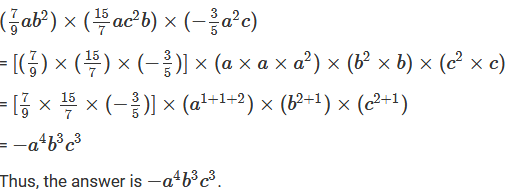Question: 14

Find the products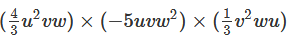Solution:

To multiply algebraic expressions, we can use commutative and associative laws along with the law of indices, am × an = am + n, wherever applicable.

We have:Question: 15

Find the productsSolution:

To multiply algebraic expressions, we can use commutative and associative laws along with the law of indices, am × an = am + n, wherever applicable.

We have: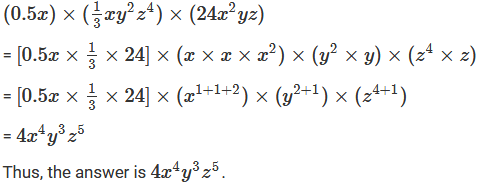Question: 16

Find the products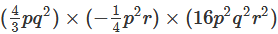Solution:

To multiply algebraic expressions, we can use commutative and associative laws along with the law of indices, am × an = am + n, wherever applicable.

We have:Question: 17

Find the products

(2.3xy) × (0.1x) × (0.16)

Solution:

To multiply algebraic expressions, we can use commutative and associative laws along with the law of indices, am × an = am + n, wherever applicable.

We have:

(2.3xy) × (0.1x) × (0.16)

= (2.3 × 0.1 × 0.16) × (x × x) × y

= (2.3 × 0.1 × 0.16) × (x1+1) × y

= 0.0368x2y

Question: 18

Express the products as a monomials and verify the result for x = 1

(3x) × (4x) × (−5x)

Solution:

To multiply algebraic expressions, we can use commutative and associative laws along with the law of indices, am × an = am + n, wherever applicable.

We have: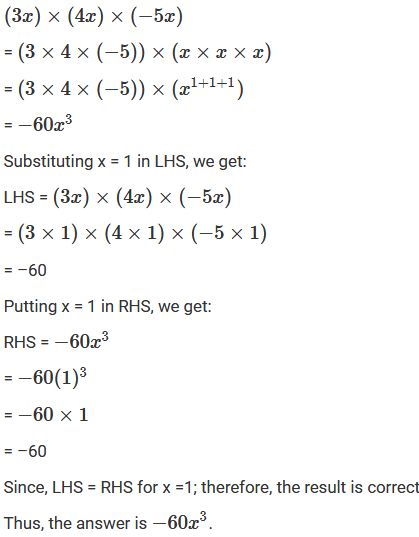Question: 19

Express the products as a monomials and verify the result for x = 1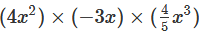Solution:

To multiply algebraic expressions, we can use commutative and associative laws along with the law of indices, am × an = am + n, wherever applicable.

We have: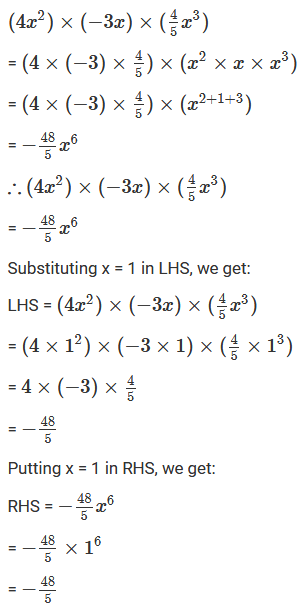Since, LHS = RHS for x = 1; therefore, the result is correct

Thus, the answer is – (48/5) x6.

Question: 20

Express the products as a monomials and verify the result for x = 1

(5x4) × (x2)3 × (2x)2

Solution:

To multiply algebraic expressions, we can use commutative and associative laws along with the law of indices, am × an = am + n, wherever applicable.

We have: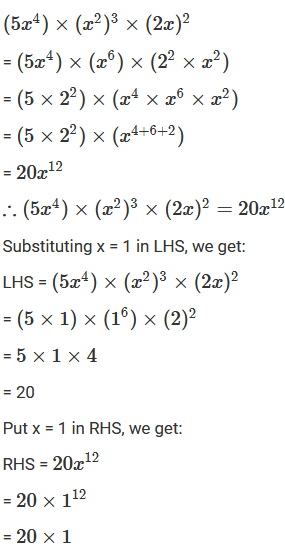Since, LHS = RHS for x = 1; therefore, the result is correct

Question: 21

Express the products as a monomials and verify the result for x = 1

(x2)3 × (2x) × (−4x) × (5)

Solution:

To multiply algebraic expressions, we can use commutative and associative laws along with the law of indices, am × an = am + n, wherever applicable.

We have:Since, LHS = RHS for x = 1; therefore, the result is correct

Question: 22

Write down the product of −8x2y6 and −20xy. Verify the product for x = 2.5, y = 1.

Solution:

To multiply algebraic expressions, we can use commutative and associative laws along with the law of indices, am × an = am + n, wherever applicable.

We have:Because LHS is equal to RHS, the result is correct.

Question: 23

Evaluate (3.2x 6y3) × (2.1x2y2) when x = 1 and y = 0.5.

Solution:

To multiply algebraic expressions, we can use commutative and associative laws along with the law of indices, am × an = am + n, wherever applicable.

We have:Question: 24

Find the value of (5x6) × (−1.5x2y3) × (−12xy2) when x = 1, y = 0.5.

Solution:

To multiply algebraic expressions, we can use commutative and associative laws along with the law of indices, am × an = am + n, wherever applicable.

We have: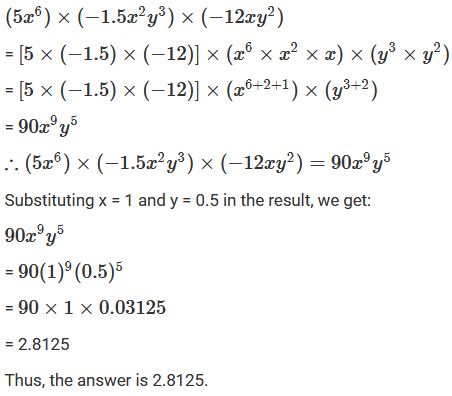Question: 25

Evaluate when (2.3a5b2) × (1.2a2b2) when a = 1 and b = 0.5.

Solution:

To multiply algebraic expressions, we can use commutative and associative laws along with the law of indices, am × an = am + n, wherever applicable.

We have:Question: 26

Evaluate for (−8x2y6) × (−20xy) x = 2.5 and y = 1

Solution:

To multiply algebraic expressions, we can use commutative and associative laws along with the law of indices, am × an = am + n, wherever applicable.

We have: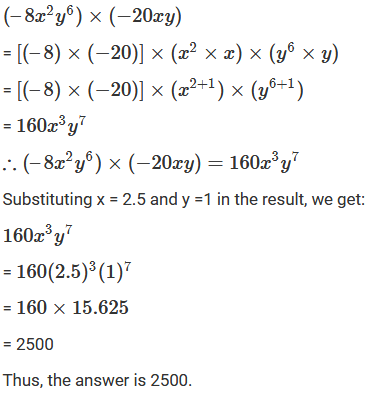Question: 27

Express the products as a monomials and verify the result for x = 1, y = 2

(-xy3) × (yx3) × (xy)

Solution:

To multiply algebraic expressions, we can use commutative and associative laws along with the law of indices, am × an = am + n, wherever applicable.

We have:Question: 28

Express the products as a monomials and verify the result for x = 1, y = 2Solution:

To multiply algebraic expressions, we can use commutative and associative laws along with the law of indices, am × an = am + n, wherever applicable.

We have: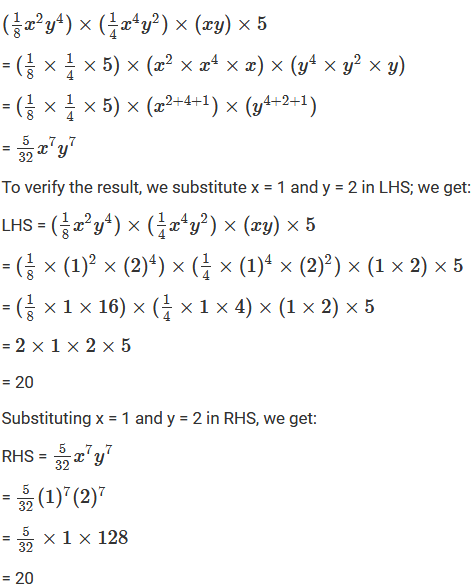Because LHS is equal to RHS, the result is correct.

Question: 29

Express the products as a monomials and verify the result for x = 1, y = 2Solution:

To multiply algebraic expressions, we can use commutative and associative laws along with the law of indices, am × an = am + n, wherever applicable.

We have: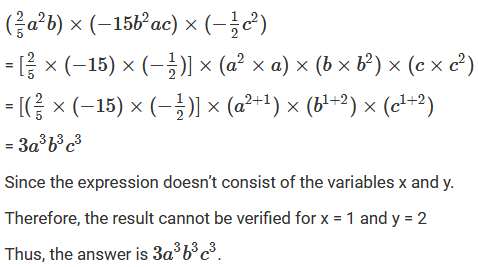Question: 30

Express the products as a monomials and verify the result for x = 1, y = 2Solution:

To multiply algebraic expressions, we can use commutative and associative laws along with the law of indices, am × an = am + n, wherever applicable.

We have:Question: 31

Express the products as a monomials and verify the result for x = 1, y = 2Solution:

To multiply algebraic expressions, we can use commutative and associative laws along with the law of indices, am × an = am + n, wherever applicable.

We have:Question: 32

Evaluate of the following when x = 2, y = -1.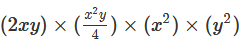Solution:

To multiply algebraic expressions, we can use commutative and associative laws along with the law of indices, am × an = am + n, wherever applicable.

We have: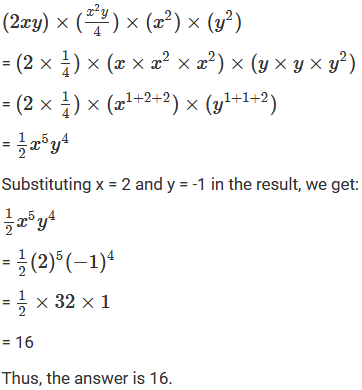Question: 33

Evaluate of the following when x = 2, y = -1.Solution:

To multiply algebraic expressions, we can use commutative and associative laws along with the law of indices, am × an = am + n, wherever applicable.

We have: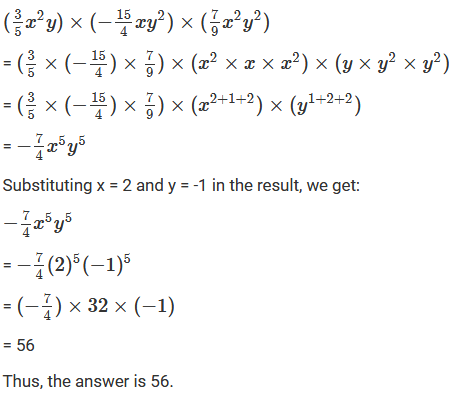### Course Features

• 728 Video Lectures
• Revision Notes
• Previous Year Papers
• Mind Map
• Study Planner
• NCERT Solutions
• Discussion Forum
• Test paper with Video Solution